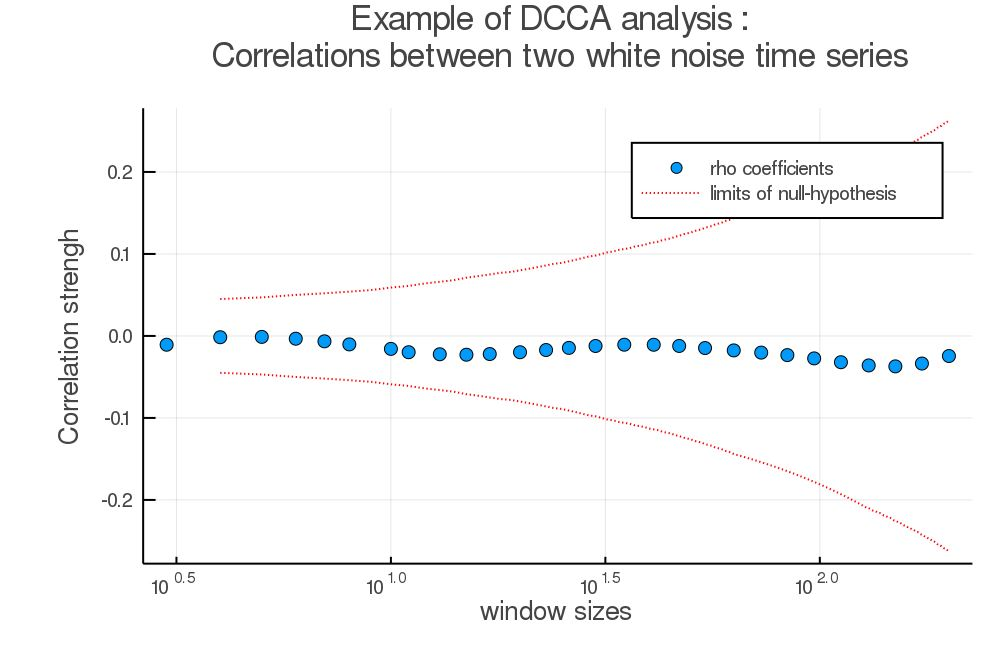## DCCA.jl

Julia module for Detrended Cross-Correlation Analysis.
Popularity
7 Stars
Updated Last
6 Months Ago
Started In
June 2018

# Detrended Cross-Correlation Analysis

A module to perform DCCA coefficients analysis. The coefficient `rho` describes the correlation strength between two time-series depending on time scales. It lies in [-1, 1], 1 being perfect correlations, and -1 perfect anticorrelations.
The package provides also functions returning a 95% confidence interval for the null-hypothesis (= "no-correlations").

The implementation is based on Zebende G, Et al. DCCA cross-correlation coefficient differentiation: Theoretical and practical approaches (2013), and was tested by reproducing the results of DCCA and DMCA correlations of cryptocurrency markets (2020) from Paulo Ferreira, et al.

## Perform a DCCA coefficients computation:

To compute DCCA coefficients, call the `rhoDCCA` function like: `pts, rho = rhoDCCA(timeSeries1, timeSeries2)`. It has the following parameters:

`rhoDCCA(timeSeries1, timeSeries2; box_start = 3, box_stop = div(length(series1),10), nb_pts = 30, order = 1)`

Input arguments:

• timeSeries1, timeSeries2 (Array{Float64,1}): Time series to analyse, need to be of the same length.
• box_start, box_stop (Int): Start and end point of the analysis. defaults respectively to 3 (the minimal possible time-scale) and 1/10th of the data length (passed this size the variance gets large).
• nb_pts (Int): Number of points to carry the analysis onto. mostly relevant for plotting.
• order (Int): Order of the polynomial to use for detrending. If not given, defaults to 1 (linear detrending). If `order` is too high, overfitting can happen, impacting the results.

Returns:

• pts (Array{Int,1}): List of points (time-scales) where the analysis is carried out.
• rho (Array{Float64,1}): Value of the DCCA coefficient at each points in `pts`.

## Get the 95% confidence interval

As a rule of thumb : values of `rho` in [-0.1,0.1] usually aren't significant.

The confidence intervals provided by this package correspond to the null-hypothesis i.e no correlations. If `rho` gets outside of this interval it can be considered significant.

To get a fast estimation of the confidence interval, call the `empirical_CI` function like: `pts, ci = empirical_CI(dataLength)`.

For a more accurate estimation, you can call `bootstrap_CI`: `pts, ci = bootstrap_CI(timeSeries1, timeSeries2; iterations = 200)`. This operation can be much more demanding (a few minutes). The `iterations` argument controls the number of repetitions for the bootstrap procedure, the higher the value, the smoother and cleaner the estimation will be, but it will also take longer.

## Example of simple analysis:

Calling the DCCA function with random white noise

```julia> ts1 = rand(2000)
ts2 = rand(2000)
x, y = rhoDCCA(ts1, ts2)
pts, ci = empirical_CI(length(ts1))```

Gave the following plot :

```a = scatter(x,y, markersize = 7, xscale = :log, title = "Example of DCCA analysis : \n Correlations between two white noise time series", label = "rho coefficients", xlabel = "window sizes", ylabel = "Correlation strengh")
plot!(a,pts,ci, color = "red", linestyle = :dot, label = "limits of null-hypothesis")
plot!(a,pts,-ci, color = "red", linestyle = :dot, label = "")
display(a)```As noted previously, the value here lies in [-0.1,0.1] although we took here 2 series of white uncorrelated noise.

## Installation:

```julia> Using Pkg